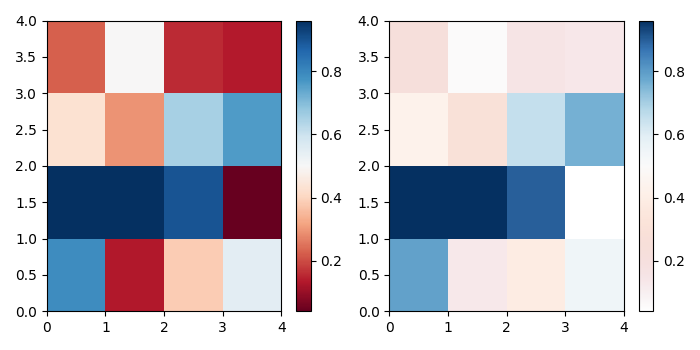# Add alpha to an existing Matplotlib colormap

To add apha to an existing matplotlib colormap, we can take the following steps −

• Create data with a 4×4 dimension array using numpy.

• Get the colormap using plt.cm.RdBU.

• Create a new colormap using numpy.

• Set alpha value to the new colormap.

• Generate a colormap object using the list of colors.

• Create a new figure or activate an existing figure using figure() method.

• Add a subplot to the current figure, nrows=1, ncols=2 at index=1.

• Create a pseudocolor plot with a non-regular rectangular grid using pcolormesh() method.

• Create a colorbar for scalar mappable instance.

• Repeat steps 7 to 9, at index 2.

• Use tight_layout() to adjust the padding between and around the subplots.

• To display the figure, use show() method.

## Example

import numpy as np
from matplotlib import pyplot as plt
from matplotlib.colors import ListedColormap
plt.rcParams["figure.figsize"] = [7.00, 3.50]
plt.rcParams["figure.autolayout"] = True
data1 = np.random.random((4, 4))
cmap = plt.cm.RdBu
my_cmap = cmap(np.arange(cmap.N))
my_cmap[:, -1] = np.linspace(0, 1, cmap.N)
my_cmap = ListedColormap(my_cmap)
plt.figure()
plt.subplot(121)
plt.pcolormesh(data1, cmap=plt.cm.RdBu)
plt.colorbar()
plt.subplot(122)
plt.pcolormesh(data1, cmap=my_cmap)
plt.colorbar()
plt.tight_layout()
plt.show()

## Output